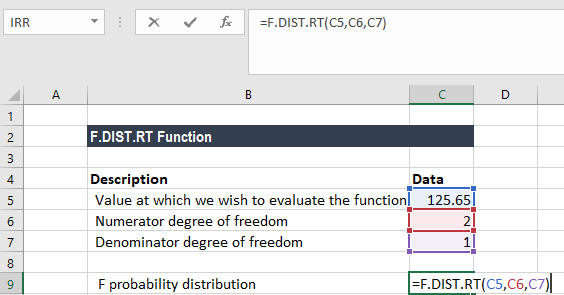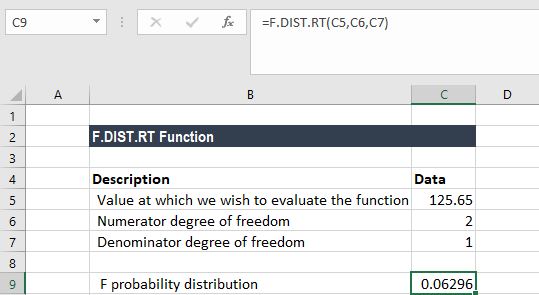# F.DIST.RT Function

Calculates the (right-tailed) F Probability Distribution

## What is the F.DIST.RT Function?

The F.DIST.RT Function is categorized under Excel Statistical functions. It will calculate the (right-tailed) F probability distribution. It measures the degree of diversity between two data sets.

For example, we can use the function if we wish to find out the variability in the test scores of female employees in the company’s finance department vis-à-vis male employees.

As a financial analyst, F.DIST.RT is useful in risk management, specifically in understanding and evaluating the extent of losses a portfolio can suffer.

The F.DIST.RT was introduced in MS Excel 2010, replacing the FDIST function.

### Formula

=F.DIST.RT(x,deg_freedom1,deg_freedom2)

The F.DIST.RT function uses the following arguments:

1. X (required argument) – This is the value at which we evaluate the function.
2. Deg_freedom1 (required argument) – This is an integer specifying the numerator degrees of freedom.
3. Deg_freedom2 (required argument) – An integer specifying the denominator degrees of freedom.

F.DIST.RT is calculated as F.DIST.RT=P(F>x), where F is a random variable with an F distribution and deg_freedom1 and deg_freedom2 as degrees of freedom.

### How to use the F.DIST.RT Function in Excel?

To understand the uses of the F.DIST.RT function, let’s consider an example:

#### Example

Suppose we are given the following data:

• Value at which we wish to evaluate the function: 65
• Numerator degree of freedom: 2
• Denominator degree of freedom: 1

To find out the F probability distribution using the F.DIST.RT function, we will use the following formula:We get the result below:### Things to remember about the F.DIST.RT Function

1. If either deg_freedom1 or deg_freedom2 is a decimal number, it is truncated to an integer by MS Excel.
2. #NUM! error – Occurs if either:
1. The value of x provided is less than 0 (negative).
2. The argument deg_freedom1 or deg_freedom2 is less than 1.
3. #VALUE! error – Occurs when any of the arguments provided is non-numeric.
4. The F.DIST.RT function is available only in MS Excel 2010 and subsequent versions.

Thanks for reading CFI’s guide to important Excel functions! By taking the time to learn and master these functions, you’ll significantly speed up your financial analysis. To learn more, check out these additional CFI resources:

• Excel Functions for Finance